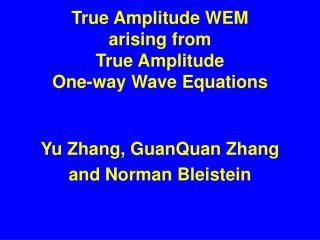# True Amplitude WEM arising from True Amplitude One-way Wave Equations - PowerPoint PPT PresentationDownload PresentationTrue Amplitude WEM arising from True Amplitude One-way Wave Equations

True Amplitude WEM arising from True Amplitude One-way Wave Equations
Download Presentation## True Amplitude WEM arising from True Amplitude One-way Wave Equations

- - - - - - - - - - - - - - - - - - - - - - - - - - - E N D - - - - - - - - - - - - - - - - - - - - - - - - - - -
##### Presentation Transcript

1. True Amplitude WEM arising from True AmplitudeOne-way Wave Equations Yu Zhang, GuanQuan Zhang and Norman Bleistein

2. Basic Plan • Derive dynamically correct one-way wave equations. • Migrate data with the new equations. • Apply imaging condition to dynamically correct migrated data. • Show computer output.

3. Forward modeling Constant wave speed Fourier transform in t, x, y

4. What happens when ? • Kinematics are correct. • Dynamics are not.

5. G. Q. Zhang  Theorem: It works! • Same eikonal equations • Same transport equation

6. Wave equation migration

7. Imaging condition

8. True amplitude WEM

9. Imaging condition

10. Theorem: It Works! True Amplitude WEM T Common-shot Kirchhoff Inversion

11. Image: U/D

12. Image: pU/pD

13. Summary • True amplitude one-way wave equations with proof. • True amplitude common-shot WEM with proof. • Numerical check for v(z) only.